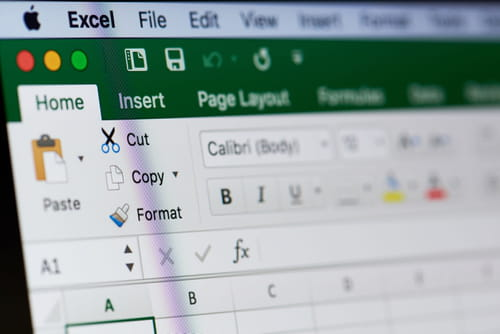14
Thanks

A few words of thanks would be greatly appreciated.

# Nesting More Than 7 IF Statements in an Excel Function

In normal circumstances, Excel places a limit on the number of nested conditional formulas that you can use. The limit is 7. However, it is possible to circumvent the limitation over the number of nested conditional formulas by cascading them.## Nesting Multiple IF Statements Using Text Data)

Consider cell A2 as the source cell, in which you will either enter a text or numeric value. Take as an example data in text format ranging from "one" to "fifty-six". To facilitate the monitoring data that will be collected in the numeric format and inserted into the formula, they could be located in target cells, in this case referring to the address of each cell.

• Simply complete the value if false of each nest by 0 (zero) and restart a new nesting, preceded by +:
`=IF(A2="one",1,IF(A2="two",2,IF(A2="three",3,IF(A2="four",4,IF(A2="five",5,IF(A2="six",6,IF(A2="seven",7,0)))))))+IF(A2="eight",... `

## Nesting Multiple IF Statements Using Numeric Data)

This time, consider cell A8 as the source cell. The data will be in numeric format, ranging from 1 to 40, and the data in the cell (e.g. D8) in text format.

• Simply complete the value if false for each nests by "" (empty), and restart a nesting preceded by an &:
`=IF(A8=1,"one",IF(A8=2,"two",IF(A8=3,"three",IF(A8=4,"four",IF(A8=5,"five",IF(A8=6,"six",IF(A8=7,"seven","")))))))&IF(A8=8,...`

## Nesting Multiple IF Statements Using Fields

This method allows you to create fields containing the conditional formula. First, enable the cell that will display the result (e.g cell D5).

• Create the first field. In refer to, enter the formula and finish with classical conditional Value if false = FALSE:
`=IF(Sheet1!A2="one",1,IF(Sheet1!A2="two",2,IF(Sheet1!A2="three",3,IF(Sheet1!A2="four",4,IF(Sheet1!A2="five",5,IF(Sheet1!A2="six",6,IF(Sheet1!A2="seven",7,FALSE)))))))`
• Continue by creating a new field named Form2, and enter the following formula to continue the conditional nesting:
`=IF(Sheet1!A2="eight",8,IF(Sheet1!A2="nine",9,IF(Sheet1!A2="ten",10,IF(Sheet1!A2="eleven",11,IF(Sheet1!A2="twelve",12,IF(Sheet1!A2="thirteen",13,IF(Sheet1!A2="fourteen",14,FALSE)))))))`

In the active cell D5, enter a simple conditional formula referring to named fields:

`=IF(Form1,Form1,IF(Form2,Form2,IF(Form3,Form3,IF(Form4,Form4,IF(Form5,Form5,IF(Form6,Form6,IF(Form7,Form7,IF(Form8,Form8,"")))))))) `

The possibilities are very important, and we have 7 nested by field X 8 or 56 conditional IF.

It is also possible to relaunch a new cascade nest based on the same criteria, which allows for a very large number of possibilities:

`IF(Form8,Form8,""))))))))&IF(Form9,...`

or

`IF(Form8,Form8,0))))))))+IF(Form9,... `

This last method is interesting because it allows you to test multiple cells, including several sheets, or create a cascade of cells, or sheets, or in a defined order.

## Nesting Multiple IF Statements Using Masked Cells

This method will allow us to obtain an unlimited number of conditionals using masked cells in a column.

Example, in a first cell (C13), the first of the 6 conditional, completed by the value if false referring to the cell containing the second conditional formula C14:

`=IF(Sheet1!A11="one",1,IF(Sheet1!A11="two",2,IF(Sheet1!A11="three",3,IF(Sheet1!A11="four",4,IF(Sheet1!A11="five",5,IF(Sheet1!A11="six",6,IF(Sheet1!A11="seven",7,C14)))))))`

In cell C14, put the following conditional formula with a value if false pointing to the address of the cell containing the formula (C15):

`=IF(Sheet1!A11="eight",8,IF(Sheet1!A11="nine",9,IF(Sheet1!A11="ten",10,IF(Sheet1!A11="eleven",11,IF(Sheet1!A11="twelve",12,IF(Sheet1!A11="thirteen",13,IF(Sheet1!A11="fourteen",14,C15)))))))`

This can be used as in many cells as needed, without limitation.

## Using Single Sheets to Simply a Formula

When all the cells are tested on the same sheet, it is possible to simplify the formula, replacing Sheet1!A2 by cell address A2 or absolute reference \$A\$2.

Image: © Dzmitry Kliapitski - Shutterstock.com

14
Thanks

A few words of thanks would be greatly appreciated.